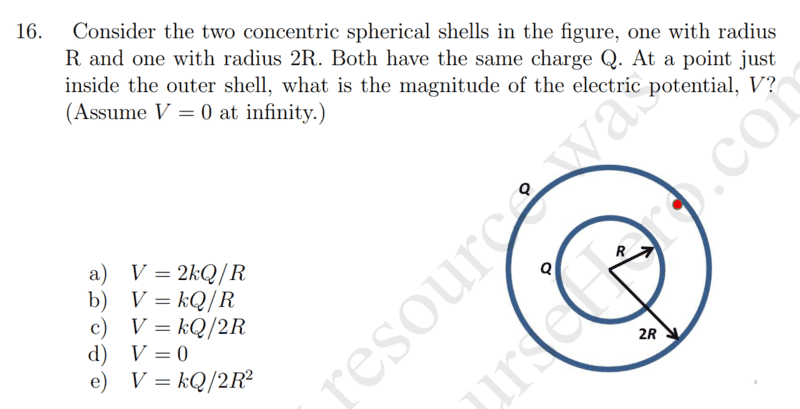# Electric Potential at Radius R of Concentric Spheres

## Homework StatementV=kQ/R

## The Attempt at a Solution

The answer is B)kQ/R. It is because V= k(2Q)/R. I don't understand why Q=2Q in this case. Isn't the point on the inside of the outer shell, so the Q for the equation is just Q?

rude man
Homework Helper
Gold Member

## Homework Statement

View attachment 231679

V=kQ/R

## The Attempt at a Solution

The answer is B)kQ/R. It is because V= k(2Q)/R. I don't understand why Q=2Q in this case. Isn't the point on the inside of the outer shell, so the Q for the equation is just Q?
The potential at any point between infinity and the outer side of the outer shell is what?
And going thru the outer shell itself to the inner side of the outer shell changes that potential by how much?

The potential at any point between infinity and the outer side of the outer shell is what?
And going thru the outer shell itself to the inner side of the outer shell changes that potential by how much?
Potential from any point between inifitiy and outer side is just V=KQ/R. Going thru the outshell to just inner side of outershell is practically zero? So, is that why it is just V=KQ/R?

rude man
Homework Helper
Gold Member
Potential from any point between inifitiy and outer side is just V=KQ/R. Going thru the outshell to just inner side of outershell is practically zero? So, is that why it is just V=KQ/R?
What is the distance in this case? What is the total charge?

Last edited:
What is the distance in this case? What is the total charge?
I am confused. What distance you mean?

rude man
Homework Helper
Gold Member
I am confused. What distance you mean?
The distance d in the formula for potential kQ/d. Or, to find the potential by integrating the E field, what are the limits of integration?

The distance d in the formula for potential kQ/d. Or, to find the potential by integrating the E field, what are the limits of integration?
I think i understand. First, integrate from infinity to 2R which is K2Q/(2R). Then integrate from outer to inner surface of the outer shell, it would be 0 because E=0 inside the shell since V integeates over the efield which is just zer0. So the potential is KQ/r since the Qs cancel.

rude man
Homework Helper
Gold Member
I think i understand. First, integrate from infinity to 2R which is K2Q/(2R). Then integrate from outer to inner surface of the outer shell, it would be 0 because E=0 inside the shell since V integeates over the efield which is just zer0. So the potential is KQ/r since the Qs cancel.
Straight A!